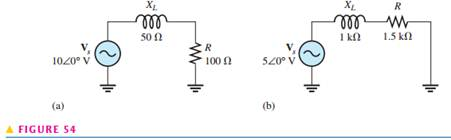Create an Account

Home / Questions / Express the total impedance of each circuit in Figure 54 in both polar and rectangular forms

Express the total impedance of each circuit in Figure 54 in both polar and rectangular forms

Express the total impedance of each circuit in Figure 54 in both polar and rectangular forms.Jul 31 2020 View more View LessSubscribe To Get Solution